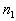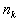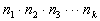TitleCollege Algebra
Tutorial 55: Fundamental Counting PrincipleLearning Objectives

 After completing this tutorial, you should be able to: Use the Fundamental Counting Principle to determine the number of outcomes in a problem.Introduction

 In this tutorial we will be going over the Fundamental Counting Principle.  It will allow us to count the number of ways a task can occur given a series of events.  Basically you multiply the number of possibilities each event of the task can occur.   It is like multiplying the dimensions of it.  I think you are ready to count away.Tutorial

 Basic Counting Principle

 Suppose that a task involves a sequence of k choices.  Letbe the number of ways the first stage or event can occur andbe the number of ways the second stage or event can occur after the first stage has occurred.  Continuing in this way, letbe the number of ways the kth stage or event can occur after the first k - 1 stages or events have occurred.  Then the total number of different ways the task can occur is:Example 1:    A deli has a lunch special which consists of a sandwich, soup, dessert and drink for \$4.99.  They offer the following choices: Sandwich: chicken salad, ham, and tuna, and roast beef Soup:  tomato, chicken noodle, vegetable Dessert: cookie and pie Drink: tea, coffee, coke, diet coke and sprite How many lunch specials are there?

Let’s use the basic counting principle:

There are 4 stages or events: choosing a sandwich, choosing a soup, choosing a dessert and choosing a drink.

There are 4 choices for the sandwich, 3 choices for the soup, 2 choices for the dessert and 5 choices for the drink

Putting that all together we get:

 Sand. Soup Dessert Drink # of lunch specials 4 x 3 x 2 x 5 = 120

So there are 120 lunch specials possible.Example 2:    You are taking a test that has five True/False questions.  If you answer each question with True or False and leave none of them blank, in how many ways can you answer the whole test?

Let’s use the basic counting principle:

There are 5 stages or events: question 1, question 2, question 3, question 4, and question 5.

There are 2 choices for each question.

Putting that all together we get:

 quest. 1 quest. 2 quest. 3 quest. 4 quest. 5 # of ways to answer test 2 x 2 x 2 x 2 x 2 = 32

So there are 32 different ways to answer the whole test.Example 3:    A company places a 6-symbol code on each unit of product.  The code consists of 4 digits, the first of which is the number 5,  followed by 2 letters, the first of which is NOT a vowel.  How many different codes are possible?

Let’s use the basic counting principle:

There are 6 stages or events: digit 1, digit 2, digit 3, digit 4, letter 1, and letter 2.

In general there are 10 digits: 0, 1, 2, 3, 4, 5, 6, 7, 8, and 9.  The first digit is limited to being the number 5, so there is only one possibility for that one.  There are no restriction on digits 2 - 4, so each one of those has 10 possibilities.

In general, there are 26 letters in the alphabet. The first letter, cannot be a vowel (a, e, i, o, u), so that means there are 21 possible letters that could go there.  The second letter has no restriction, so there are 26 possibilities for that one.

Putting that all together we get:

 digit 1 digit 2 digit 3 digit 4 letter 1 letter 2 # of codes 1 x 10 x 10 x 10 x 21 x 26 = 546000

So there are 546000 different 6-symbol codes possible.Practice Problems

 These are practice problems to help bring you to the next level.  It will allow you to check and see if you have an understanding of these types of problems. Math works just like anything else, if you want to get good at it, then you need to practice it.  Even the best athletes and musicians had help along the way and lots of practice, practice, practice, to get good at their sport or instrument.  In fact there is no such thing as too much practice. To get the most out of these, you should work the problem out on your own and then check your answer by clicking on the link for the answer/discussion for that  problem.  At the link you will find the answer as well as any steps that went into finding that answer.Practice Problems 1a - 1c: Solve using the counting principle.

 1a.  One quarter, one dime and one six-sided die are tossed.  How many results are possible? (answer/discussion to 1a)

 1b.  Next semester you are going to take one science class, one math class, one history class and one english class.  According to the schedule you have 4 different science classes, 3 different math classes, 2 different history classes, and 3 different English classes to choose from.  Assuming no scheduling conflicts, how many different four-course selections can you make? (answer/discussion to 1b)

 1c.  Six students in a speech class all have to give there speech on the same day.  One of the students insists on being first.  If this student’s request is granted, how many different ways are there to schedule the speeches? (answer/discussion to 1c)Need Extra Help on these Topics?

 Go to Get Help Outside the Classroom found in Tutorial 1: How to Succeed in a Math Class for some more suggestions.

Last revised on May 19, 2011 by Kim Seward.
All contents copyright (C) 2002 - 2011, WTAMU and Kim Seward. All rights reserved.